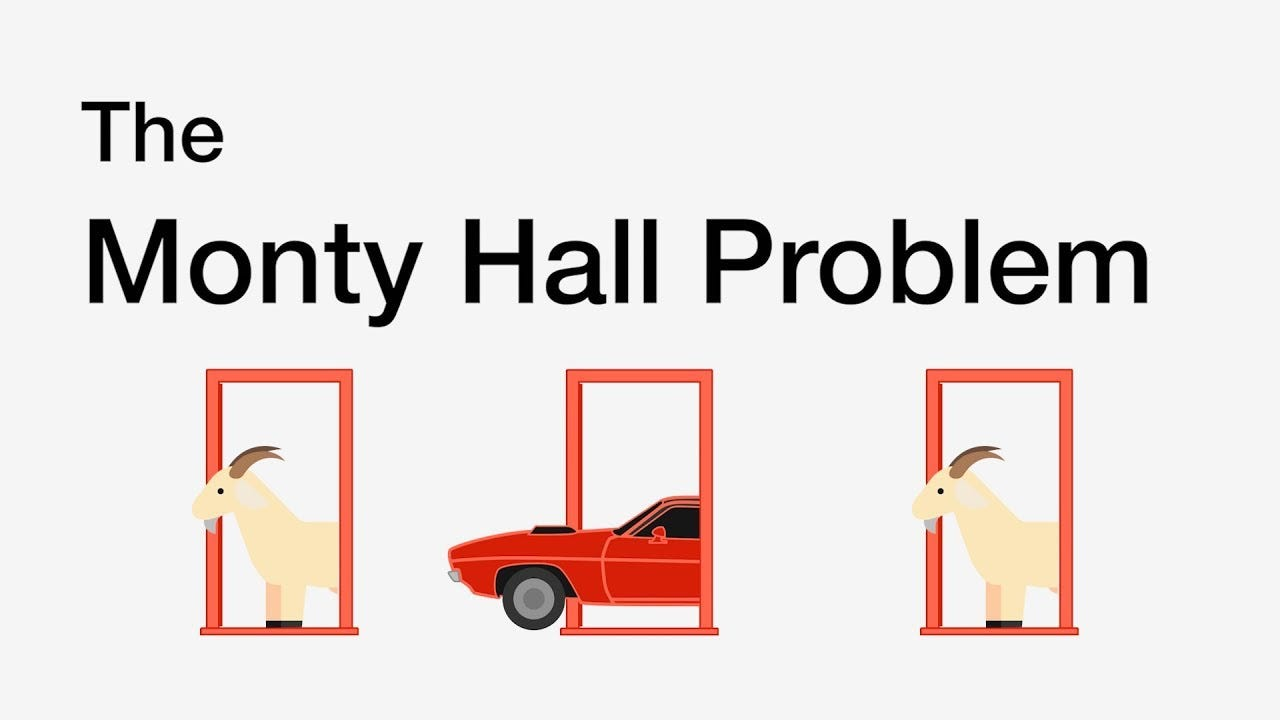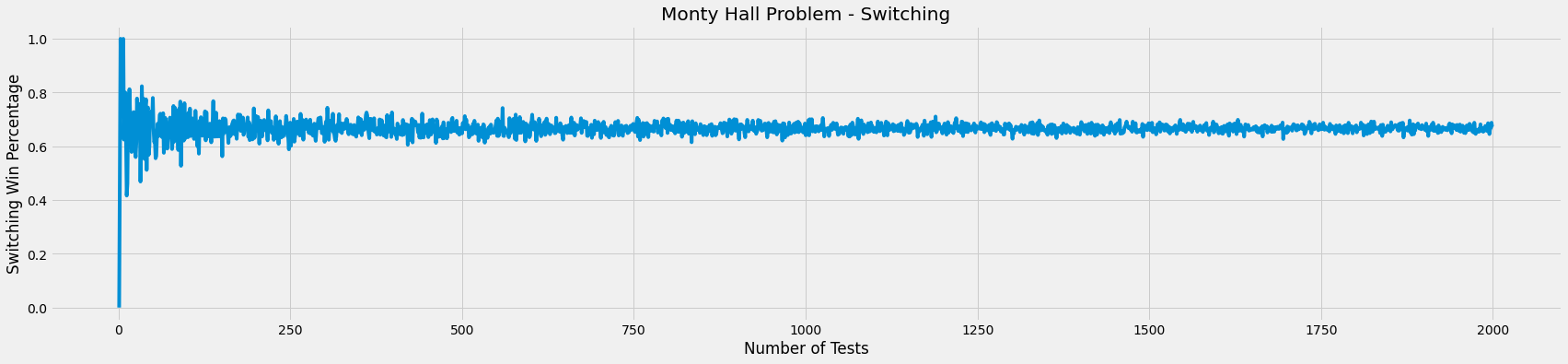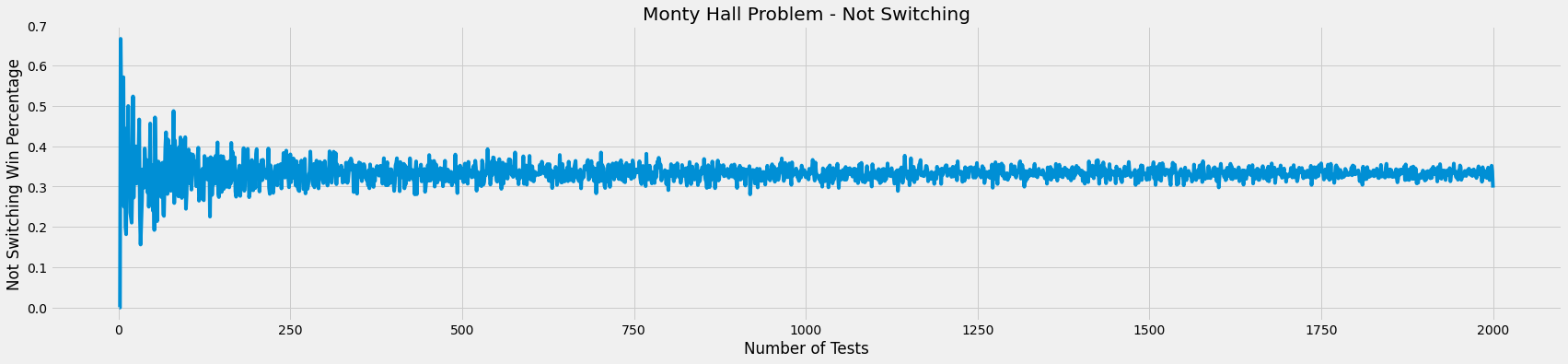# The Monty Hall Problem¶Image from medium.com

The Monty Hall problem is named for its similarity to the Let's Make a Deal television game show hosted by Monty Hall. The problem is stated as follows. Assume that a room is equipped with three doors. Behind two are goats, and behind the third is a shiny new car. You are asked to pick a door, and will win whatever is behind it. Let's say you pick door 1. Before the door is opened, however, someone who knows what's behind the doors (Monty Hall) opens one of the other two doors, revealing a goat, and asks you if you wish to change your selection to the third door (i.e., the door which neither you picked nor he opened). The Monty Hall problem is deciding whether you do.

In :
import random
import matplotlib.pyplot as plt
plt.style.use('fivethirtyeight')

In :
# Create a function for the host to reveal a door that doesn't contain the prize and a door that isn't the contenstants original choice
def get_non_prize_door(host, num_doors, player_choice):
i = 1
while (i == host or i == player_choice):
i = (i + 1) % (num_doors)
return i

In :
# Create a function to have the player switch to the other unopened door.
def switch_function(shown_door, num_doors, player_choice):
i = 1
while (i == shown_door or i == player_choice):
i = (i + 1) % (num_doors)
return i

In :
# Create a function to simulate the game
def monty_hall_game(switch, num_tests):

win_switch_count = 0
win_no_switch_count = 0
lose_switch_count = 0
lose_no_switch_count = 0

doors = [0, 1, 2]
num_doors = len(doors)

# Loop through the number of times the contestant can play the game
for i in range(0, num_tests):
door_with_prize = random.randint(0, num_doors - 1)
host = door_with_prize
player_choice = random.randint(0, num_doors - 1)
original_player_choice = player_choice
shown_door = get_non_prize_door(host, num_doors, player_choice)

# If the contestant choose to always switch then allow the player to switch their original chosen door to the other unopened door
if switch == True:
player_choice = switch_function(shown_door, num_doors, player_choice)

if player_choice == door_with_prize and switch == False:
# Then the player wins from not switching
win_no_switch_count = win_no_switch_count + 1

elif player_choice == door_with_prize and switch == True:
# Then the player wins from switching
win_switch_count = win_switch_count + 1

elif player_choice != door_with_prize and switch == False:
# Then the player lost from not switching
lose_no_switch_count = lose_no_switch_count + 1

elif player_choice != door_with_prize and switch == True:
# Then the player lost from switching
lose_switch_count = lose_switch_count + 1

return win_no_switch_count, win_switch_count, lose_no_switch_count, lose_switch_count, num_tests

In :
# Play the game!
x = monty_hall_game(True, 100);

In :
# Get the win / lose percenage for switch & not switching
print("Win switch %: ", x / x)
print("Lose switch %: ", x / x)

Win switch %:  0.67
Lose switch %:  0.33

In :
# Play the game!
x = monty_hall_game(False, 100);

In :
print("Win no switch %: ", x / x)
print("Lose no switch %: ", x / x)

Win no switch %:  0.33
Lose no switch %:  0.67

In :
# Get the data to create a visualization of the number of simulated tests / games played
num_tests = []
s_win_percentage = []
switch = True
# Run up to 2000 simulated games
for i in range(1, 2001):
num_tests.append(i)
y = monty_hall_game(switch, i)
s_win_percentage.append(y / y)

In :
# Visually show the number of tests and the win percentage from always switching
plt.figure(figsize = (26.2, 5.5))
plt.plot(num_tests, s_win_percentage)
plt.xlabel('Number of Tests')
plt.ylabel('Switching Win Percentage')
plt.title("Monty Hall Problem - Switching")
plt.show()In :
print("The win percentage for switching on", y, "games is:", round(y/y*100, 2),"%")

The win percentage for switching on 2000 games is: 67.1 %

In :
# Get the data to create a visualization of the number of simulated tests / games played
num_tests = []
nw_win_percentage = []
switch = False
# Run up to 2000 simulated games
for i in range(1, 2001):
num_tests.append(i)
y = monty_hall_game(switch, i)
nw_win_percentage.append(y / y)

In :
# Visually show the number of tests and the win percentage from always switching
plt.figure(figsize = (26.2, 5.5))
plt.plot(num_tests, nw_win_percentage)
plt.xlabel('Number of Tests')
plt.ylabel('Not Switching Win Percentage')
plt.title("Monty Hall Problem - Not Switching")
plt.show()In :
print("The win percentage for switching on", y, "games is:", round(y/y*100, 2),"%")

The win percentage for switching on 2000 games is: 29.75 %